# Calculation Rules for Listed Derivatives

## Exchange fee and registration fee

The fee schedule is defined considering the family of products. For each family, there are base contracts defined, whose average number of contracts traded will be used in the calculation of fees for all contracts in the family.

The ADV is calculated every last business day of each week, based on that and the previous 20 trading sessions, totalling an average of 21 trading sessions. This value will be used for each family of produtcts throughout the following week.

For participants in the same master account, the considered ADTV will be the sum of all participants.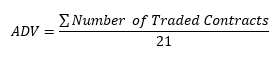For contracts that have term (Interest Rate in Reais, Interest Rate in Dollar and Interest Rate Indexed to Inflation) the volume traded must be adjusted to the duration of the contract.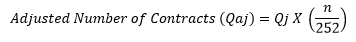Where:

Qaj = Adjusted number of contracts on maturity j;

Qj = Effective number of contracts traded on maturity j;

n = Number of reserve days between the trade date and maturity date.

For minicontracts (including minicontracts of options on U.S. dollar), the number of contracts must be divided by 5. For rollovers, the number of contracts must be multiplied by 2. For microcontracts, the number of contracts must be divided by 20.

Rounded in zero decimal places.

There is no difference between regular or daytrade operations.

If there is no trading for the base contracts in the family, the ADTV will be 1.

On the first week of trading, the ADTV will be alocated on the first tier of the table.

OBS: For HFT investors, the ADTV must be calculated according to the defined calculation methodology.

### Average cost calculation (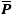)

With the ADTV from the base contracts, the next step is to calculate the average cost () for the exchange fee and variable registration fee. This factor is calculated in a progressive way, as shown below:

Progressive table of discounts
Lower limit Upper limit Tier value
D1 U1 V1
D2 U2 V2
D3 U3 V3
... ... ...
Di-1 Ui-1 Vi-1
Di Ui Vi
Dn Un Vn

The average cost for the exchange fee and variable registration fee is defined as: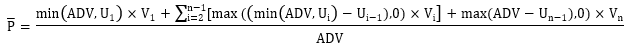Where:= calculated average cost;

ADV = average number of traded contracts, as calculated on the previous item;

Ui = Upper limit of each tier;

Un = Upper limit of the last tier;

Vi = Table value associated to each tier;

Vn = Table value associated to the last tier;

Rounding is made to the seventh decimal place for contracts that have term (Interest Rate in Reais, Interest Rate in Dollar, Interest Rate Indexed to Inflation). For all other contracts, the rounding is made to the second decimal place.

OBS: For HFT investors, the average cost is calculated on a regressive way, meaning that the fee applied to all contracts is the fee of the lowest tier reached.

### Unitary cost calculation

The unitary cost is calculated by applying the average cost obtained on the previous item to the formulas for each family of product.

The average cost is applied to the formula, as other factors needed, and the unitary coast is calculated.

Rounded to the second decimal place.

### Incentive policies application

The incentive policies are applied to the unitary cost of the exchange fee and the variable registration fee, depending on the type of operation.

Rounded to the second decimal place.

### Unitary cost calculation for exchange fee and variable registration fee

After the application of the incentive policies, the exchange fee and the registration fee are calculated, trade by trade.

#### Registration Fee

The fixed registration fee is the same to all products, except for the BRICs contracts and the ones that are exempt, according to the fee pages of each family of products.

The fixed registration fee (with seven decimal places) is added to the variable registration fee. Then, they are multiplied by the number of contracts of each trade.

Rounded to the second decimal place.

#### Exchange fee

Unitary cost for the exchange fee, multiplied by the number of contracts of each trade.

Rounded to the second decimal place.

## Permanence Fee

Considers the open interest of each product, regardless of maturity, for each account, according to the fee pages of each family of products.

The apuration period is from the last business day of the previous month to the penultimate day of hte current month. It is calculated daily and charged in the following conditions:

• Last business day of each month;
• Next day of the closure of all positions for the same product and account;
• As of the total transference of the position (account) of each product to other participant.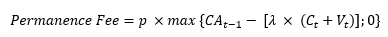Where:

p = Daily value for the permanence fee for each product;

CAt-1 = Open interest of each contract on the previous day of the calculation;

Ct + Vt = Sum of all buys and sells (not the netting) of each product traded on the day of calculation;

λ = Reduction factor.

Rounded to the second decimal place.

For contracts with permanence fee in other currencies or commodities contracts, operated by 2687 investors (non-resident investores with CVM document), the permanence fee will be converted to BRL by the Exchange Rate from the last business day of the previous month, rounded in three decimal places.

## Settlement fee

The settlement fee is a fixed value per contract, differentiated per product. It is multiplied by the number of contracts settled, rounded to the second decimal place.